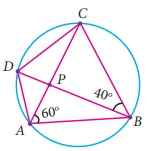Home | | Maths 9th std | Exercise 4.4: Cyclic Quadrilaterals

## Chapter: 9th Maths : UNIT 4 : Geometry

Maths : Geometry: Book Back, Exercise, Example Numerical Question with Answers, Solution : Exercise 4.4: Cyclic Quadrilaterals

Exercise 4.4

1. Find the value of x in the given figure.2. In the given figure, AC is the diameter of the circle with centre O. If ADE = 30° ; DAC = 35° and CAB = 40° .

Find (i) ACD (ii) ACB (iii) DAE3. Find all the angles of the given cyclic quadrilateral ABCD in the figure.4. In the given figure, ABCD is a cyclic quadrilateral where diagonals intersect at P such that DBC = 40° and BAC = 60° find

(ii) BCD5. In the given figure, AB and CD are the parallel chords of a circle with centre O. Such that AB = 8cm and CD = 6cm. If OM AB and OL CD distance between LM is 7cm. Find the radius of the circle?6. The arch of a bridge has dimensions as shown, where the arch measure 2m at its highest point and its width is 6m. What is the radius of the circle that contains the arch?7. In figure, ABC =120º, where A,B and C are points on the circle with centre O. Find OAC ?8. A school wants to conduct tree plantation programme. For this a teacher allotted a circle of radius 6m ground to nineth standard students for planting sapplings.Four students plant trees at the points A,B,C and D as shown in figure. Here AB = 8m, CD = 10m and AB CD. If another student places a flower pot at the point P, the intersection of AB and CD, then find the distance from the centre to P.9. In the given figure, POQ = 1000 and PQR = 30º , then find RPO .Tags : Numerical Problems with Answers, Solution | Geometry | Maths , 9th Maths : UNIT 4 : Geometry
Study Material, Lecturing Notes, Assignment, Reference, Wiki description explanation, brief detail
9th Maths : UNIT 4 : Geometry : Exercise 4.4: Cyclic Quadrilaterals | Numerical Problems with Answers, Solution | Geometry | Maths